# Knowledge Base

## Solution 32491: Constructing a Matrix Using the TI-Nspire™ Family Products.

### How do I construct a matrix using TI-Nspire family products?

The syntax to construct a matrix is as follows: constructMat(Expr,Var1,Var2,numRows,numCols)=matrix

Expr = Expression
Var1 = Variable1
Var2 = Variable2
numRows = number of rows
numCols = number of columns

Follow the steps below to construct a matrix:

1) Open a calculator document
2) Press [menu] and select 7: Matrix & Vector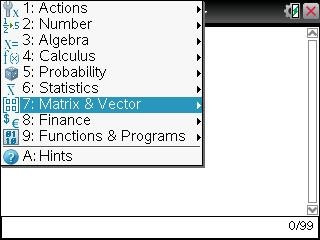3) Select 1: Create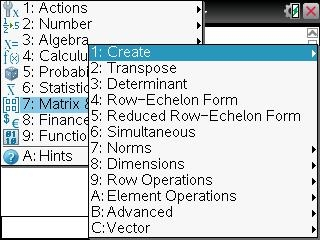4) Select A: Construct Matrix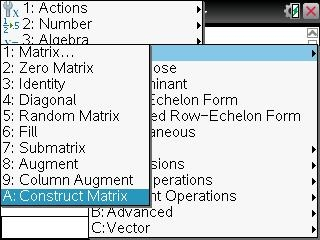5) constructMat( will display on the screen.

6) Input constructMat(x^2+6y,x,y,3,4) as shown below: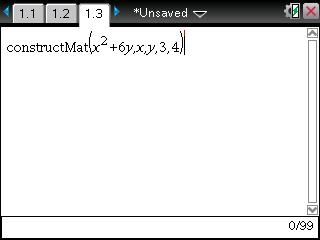7) Press [enter]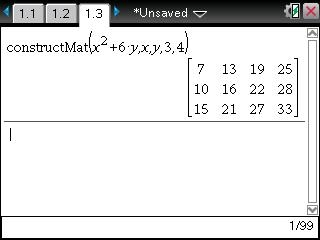Please Note: The constructMat command returns a matrix based on the arguments.

Expr is an expression in variables Var1 and Var2. Elements in the resulting matrix are formed by evaluating Expr for each incremented value of Var1 and Var2.

Var1 is automatically incremented from 1 through numRows. Within each row, Var2 is incremented from 1 through numCols.